# 价格速度测量方法

17 七月 2019, 08:27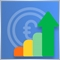0
2 302

### 价格速度测量方法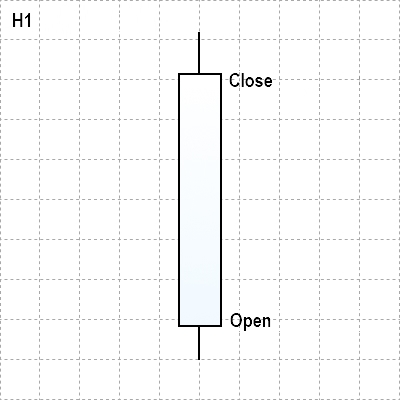• 我们定义了价格每小时通过的平均点数。然而，测量只在一根蜡烛的框架内进行，我们无法在此框架内获得总体趋势图。
• 但是，如果我们将速度从每小时的点转换为每分钟的点、5分钟的点等，我们就可以在这个H1小时内获得更有价值的价格变动数据。
• 因此，下面两个结论是显而易见的：在较低的时间段上确定速度对于较高的时间段更为方便。当通过测量价格变动来测量当前时间段的平均速度时，我们应该使用几个烛形。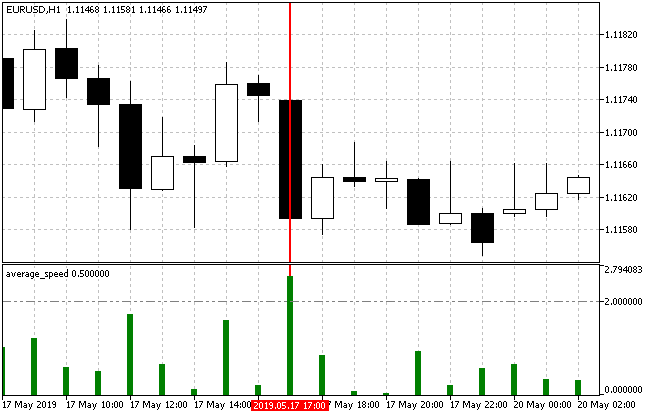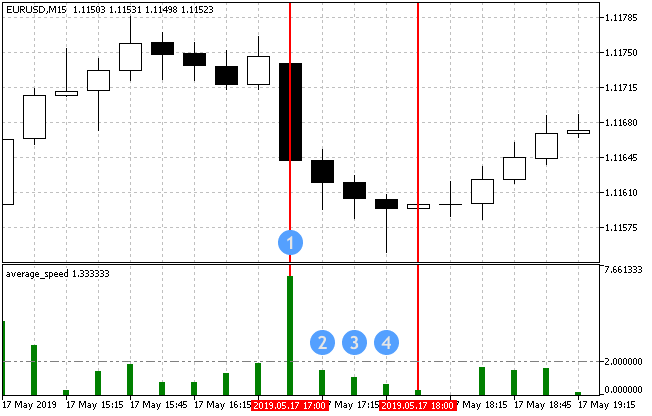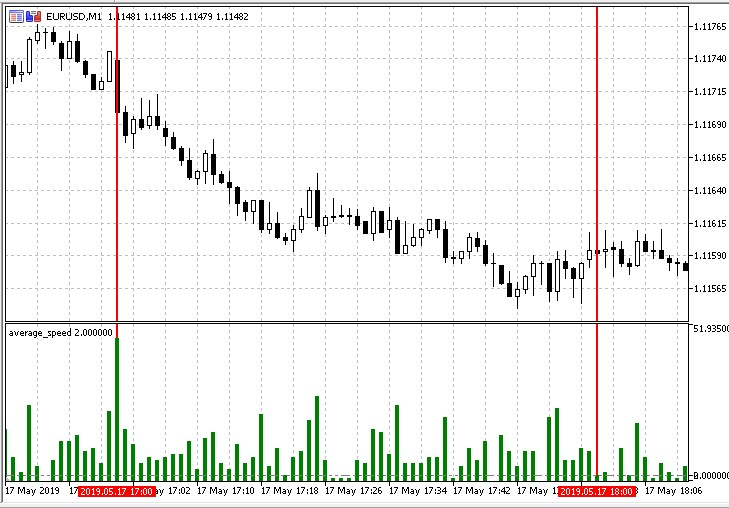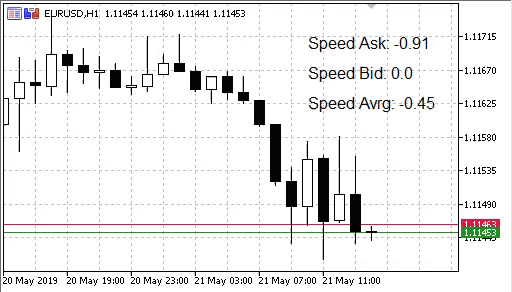• 动量指标衡量一段时间内的价格变化量。极高或较低的动量值表示当前趋势的延续。这意味着与指标的较大偏差表示当前特定方向的高价格速度。
• ADX 趋势指标. 平均定向运动指数显示了当前趋势的强度。实际上，它显示当前的平均速度。

### 基于测量方法制定交易策略

• 以单位时间内通过点的比率直接测量平均速度。
• 以通过的点数与分时数之比来测量速度。
• 使用跟随趋势的和其他指标进行间接速度测量。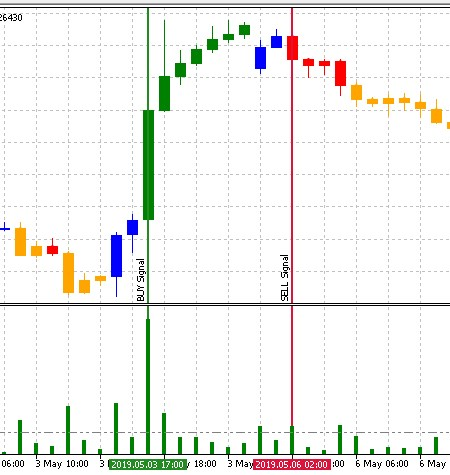```//+------------------------------------------------------------------+
//| EA 的输入参数                                                      |
//+------------------------------------------------------------------+
input string               InpEaComment         =  "Strategy #1"; // EA Comment
input int                  InpMagicNum          =  1111;          // Magic number
input double               InpLot               =  0.1;           // Lots
input uint                 InpStopLoss          =  400;           // StopLoss in points
input uint                 InpTakeProfit        =  500;           // TakeProfit in points
input uint                 InpSlippage          =  0;             // Slippage in points
input ENUM_TIMEFRAMES      InpInd_Timeframe     =  PERIOD_H1;     // Timeframe for the calculation
//--- 平均速度指标的参数
input int                  InpBars              =  1;             // Days
input ENUM_APPLIED_PRICE   InpPrice             =  PRICE_CLOSE;   // Applied price
input double               InpTrendLev          =  2;             // Trend Level
//--- CAM 指标参数
input uint                 InpPeriodADX         =  10;            // ADX period
input uint                 InpPeriodFast        =  12;            // MACD Fast EMA period
input uint                 InpPeriodSlow        =  26;            // MACD Slow EMA period
//---
CEngine        engine;
CTrade         trade;
//--- 声明指标变量和句柄
double         lot;
ulong          magic_number;
uint           stoploss;
uint           takeprofit;
uint           slippage;
int            InpInd_Handle1,InpInd_Handle2;
double         avr_speed[],cam_up[],cam_dn[];
//+------------------------------------------------------------------+
//| EA 交易初始化函数                                                   |
//+------------------------------------------------------------------+
int OnInit()
{
//--- 初始化检查
if(!TerminalInfoInteger(TERMINAL_TRADE_ALLOWED))
{
Print(InpEaComment,": 不允许进行交易!");
return(INIT_FAILED);
}
if(!TerminalInfoInteger(TERMINAL_CONNECTED))
{
Print(InpEaComment,": 没有连接!");
return(INIT_FAILED);
}
//--- 取得 Average Speed 指标句柄
InpInd_Handle1=iCustom(Symbol(),InpInd_Timeframe,"Speed Price\\average_speed",
InpBars,
InpPrice
);
if(InpInd_Handle1==INVALID_HANDLE)
{
Print(InpEaComment,": 获取 average_speed 句柄失败");
Print("Handle = ",InpInd_Handle1,"  error = ",GetLastError());
return(INIT_FAILED);
}
//--- 取得 CAM 指标句柄
InpInd_Handle2=iCustom(Symbol(),InpInd_Timeframe,"Speed Price\\CAM",
InpPeriodADX,
InpPeriodFast,
InpPeriodSlow
);
if(InpInd_Handle2==INVALID_HANDLE)
{
Print(InpEaComment,": 获取 average_speed 句柄失败");
Print("Handle = ",InpInd_Handle2,"  error = ",GetLastError());
return(INIT_FAILED);
}
//---
ArrayInitialize(avr_speed,0.0);
ArrayInitialize(cam_up,0.0);
ArrayInitialize(cam_dn,0.0);
ArraySetAsSeries(avr_speed,true);
ArraySetAsSeries(cam_up,true);
ArraySetAsSeries(cam_dn,true);
//--- 设置交易参数
lot=NormalizeLot(Symbol(),fmax(InpLot,MinimumLots(Symbol())));
magic_number=InpMagicNum;
stoploss=InpStopLoss;
takeprofit=InpTakeProfit;
slippage=InpSlippage;
//---
trade.SetDeviationInPoints(slippage);
trade.SetExpertMagicNumber(magic_number);
trade.SetTypeFillingBySymbol(Symbol());
trade.SetMarginMode();
trade.LogLevel(LOG_LEVEL_NO);
//---
return(INIT_SUCCEEDED);
}
//+------------------------------------------------------------------+
//| 计时器函数                                                         |
//+------------------------------------------------------------------+
void OnTimer()
{
if(!MQLInfoInteger(MQL_TESTER))
engine.OnTimer();
}
//+------------------------------------------------------------------+
//| EA交易分时函数                                                     |
//+------------------------------------------------------------------+
void OnTick()
{
//--- 如果在测试器中工作
if(MQLInfoInteger(MQL_TESTER))
engine.OnTimer();

if(!IsOpenedByMagic(InpMagicNum))
{
//--- 取得计算数据
if(!GetIndValue())
return;
//---
if(BuySignal())
{
//--- 取得相对止损水平的正确止损和获利价格
double sl=CorrectStopLoss(Symbol(),ORDER_TYPE_BUY,0,stoploss);
double tp=CorrectTakeProfit(Symbol(),ORDER_TYPE_BUY,0,takeprofit);
//--- 开启买入仓位
trade.Buy(lot,Symbol(),0,sl,tp);
}
else if(SellSignal())
{
//--- 取得相对止损水平的正确止损和获利价格
double sl=CorrectStopLoss(Symbol(),ORDER_TYPE_SELL,0,stoploss);
double tp=CorrectTakeProfit(Symbol(),ORDER_TYPE_SELL,0,takeprofit);
//--- 开启卖出仓位
trade.Sell(lot,Symbol(),0,sl,tp);
}
}
}
//+------------------------------------------------------------------+
//|                                                                  |
//+------------------------------------------------------------------+
bool BuySignal()
{
return(avr_speed>=InpTrendLev && cam_up!=EMPTY_VALUE)?true:false;
}
//+------------------------------------------------------------------+
bool SellSignal()
{
return(avr_speed>=InpTrendLev && cam_dn!=EMPTY_VALUE)?true:false;
}
//+------------------------------------------------------------------+
//| 取得当前指标值                                                      |
//+------------------------------------------------------------------+
bool GetIndValue()
{
return(CopyBuffer(InpInd_Handle2,0,0,1,cam_up)<1 ||
CopyBuffer(InpInd_Handle2,1,0,1,cam_dn)<1 ||
CopyBuffer(InpInd_Handle1,0,0,1,avr_speed)<1
)?false:true;
}
//+------------------------------------------------------------------+
//| 使用幻数检查开启的仓位                                               |
//+------------------------------------------------------------------+
bool IsOpenedByMagic(int MagicNumber)
{
int pos=0;
uint total=PositionsTotal();
//---
for(uint i=0; i<total; i++)
{
if(SelectByIndex(i))
if(PositionGetInteger(POSITION_MAGIC)==MagicNumber)
pos++;
}
return((pos>0)?true:false);
}
//+------------------------------------------------------------------+
//| 根据索引选择仓位                                                    |
//+------------------------------------------------------------------+
bool SelectByIndex(const int index)
{
ENUM_ACCOUNT_MARGIN_MODE margin_mode=(ENUM_ACCOUNT_MARGIN_MODE)AccountInfoInteger(ACCOUNT_MARGIN_MODE);
//---
if(margin_mode==ACCOUNT_MARGIN_MODE_RETAIL_HEDGING)
{
ulong ticket=PositionGetTicket(index);
if(ticket==0)
return(false);
}
else
{
string name=PositionGetSymbol(index);
if(name=="")
return(false);
}
//---
return(true);
}
//+------------------------------------------------------------------+```

```//+------------------------------------------------------------------+
//| EA 的输入参数                                                      |
//+------------------------------------------------------------------+
input string               InpEaComment         =  "Strategy #2"; // EA Comment
input int                  InpMagicNum          =  1111;          // Magic number
input double               InpLots              =  0.1;           // Lots
input uint                 InpStopLoss          =  400;           // StopLoss in points
input uint                 InpTakeProfit        =  500;           // TakeProfit in points
input ENUM_COPY_TICKS      tick_flags           =  TICKS_INFO;    // Ticks resulting from Bid and/or Ask changes
input int                  InpPoints            =  15;            // The price should move NNN points
input uchar                InpTicks             =  15;            // For XXX ticks

//--- 取得分时的数组
MqlTick        tick_array_curr[];            // 用于当前分时的分时数组
MqlTick        tick_array_prev[];            // 用于之前分时的分时数组
ulong          tick_from=0;                  // 如果 tick_from=0, 给出最新的 tick_count 个分时
uint           tick_count=15;                // 应当取得的分时数量
//---
double         ExtStopLoss=0.0;
double         ExtTakeProfit=0.0;
double         ExtPoints=0.0;
bool           first_start=false;
long           last_trade_time=0;
//+------------------------------------------------------------------+
//| EA 交易初始化函数                                                   |
//+------------------------------------------------------------------+
int OnInit()
{
if(!m_symbol.Name(Symbol()))              // 设置交易品种名称
return(INIT_FAILED);

tick_count+=InpTicks;                     // 请求 "tick_count" + "for XXX ticks"
ExtStopLoss=InpStopLoss*Point();
ExtTakeProfit=InpTakeProfit*Point();
ExtPoints=InpPoints*Point();
first_start=false;
//--- 请求 ticks (第一次填充)
int copied=CopyTicks(Symbol(),tick_array_curr,tick_flags,tick_from,tick_count);
if(copied!=tick_count)
first_start=false;
else
{
first_start=true;
ArrayCopy(tick_array_prev,tick_array_curr);
}
m_trade.SetExpertMagicNumber(InpMagicNum);
m_trade.SetTypeFillingBySymbol(Symbol());
m_trade.SetMarginMode();
m_trade.LogLevel(LOG_LEVEL_NO);
//---
return(INIT_SUCCEEDED);
}
//+------------------------------------------------------------------+
//| EA交易分时函数                                                     |
//+------------------------------------------------------------------+
void OnTick()
{
//--- 检查第一次开始
int copied=-1;
if(!first_start)
{
copied=CopyTicks(Symbol(),tick_array_curr,tick_flags,tick_from,tick_count);
if(copied!=tick_count)
first_start=false;
else
{
first_start=true;
ArrayCopy(tick_array_prev,tick_array_curr);
}
}
//--- 请求分时
copied=CopyTicks(Symbol(),tick_array_curr,tick_flags,tick_from,tick_count);
if(copied!=tick_count)
return;
int index_new=-1;
long last_time_msc=tick_array_prev[tick_count-1].time_msc;
for(int i=(int)tick_count-1;i>=0;i--)
{
if(last_time_msc==tick_array_curr[i].time_msc)
{
index_new=i;
break;
}
}
//---
if(index_new!=-1 && !IsOpenedByMagic(InpMagicNum))
{
int shift=(int)tick_count-1-index_new-InpTicks;   // 当前分时数组的偏移
shift=(shift<0)?0:shift;
if(tick_array_curr[tick_count-1].ask-tick_array_curr[shift].ask>ExtPoints)
{
//--- 开启 BUY
double sl=(InpStopLoss==0)?0.0:tick_array_curr[tick_count-1].ask-ExtStopLoss;
double tp=(InpTakeProfit==0)?0.0:tick_array_curr[tick_count-1].ask+ExtTakeProfit;
m_trade.Buy(InpLots,m_symbol.Name(),tick_array_curr[tick_count-1].ask,
m_symbol.NormalizePrice(sl),
m_symbol.NormalizePrice(tp));
last_trade_time=tick_array_curr[tick_count-1].time_msc;
}
else if(tick_array_curr[shift].bid-tick_array_curr[tick_count-1].bid>ExtPoints)
{
//--- 开启 SELL
double sl=(InpStopLoss==0)?0.0:tick_array_curr[tick_count-1].bid-ExtStopLoss;
double tp=(InpTakeProfit==0)?0.0:tick_array_curr[tick_count-1].bid+ExtTakeProfit;
m_trade.Sell(InpLots,m_symbol.Name(),tick_array_curr[tick_count-1].bid,
m_symbol.NormalizePrice(sl),
m_symbol.NormalizePrice(tp));
last_trade_time=tick_array_curr[tick_count-1].time_msc;
}
}
ArrayCopy(tick_array_prev,tick_array_curr);
//---
}
//+------------------------------------------------------------------+
//| 使用幻数检查开启的仓位                                               |
//+------------------------------------------------------------------+
bool IsOpenedByMagic(int MagicNumber)
{
int pos=0;
uint total=PositionsTotal();
//---
for(uint i=0; i<total; i++)
{
if(SelectByIndex(i))
if(PositionGetInteger(POSITION_MAGIC)==MagicNumber)
pos++;
}
return((pos>0)?true:false);
}
//+------------------------------------------------------------------+
//| 根据索引选择仓位                                                    |
//+------------------------------------------------------------------+
bool SelectByIndex(const int index)
{
ENUM_ACCOUNT_MARGIN_MODE margin_mode=(ENUM_ACCOUNT_MARGIN_MODE)AccountInfoInteger(ACCOUNT_MARGIN_MODE);
//---
if(margin_mode==ACCOUNT_MARGIN_MODE_RETAIL_HEDGING)
{
ulong ticket=PositionGetTicket(index);
if(ticket==0)
return(false);
}
else
{
string name=PositionGetSymbol(index);
if(name=="")
return(false);
}
//---
return(true);
}
//+------------------------------------------------------------------+```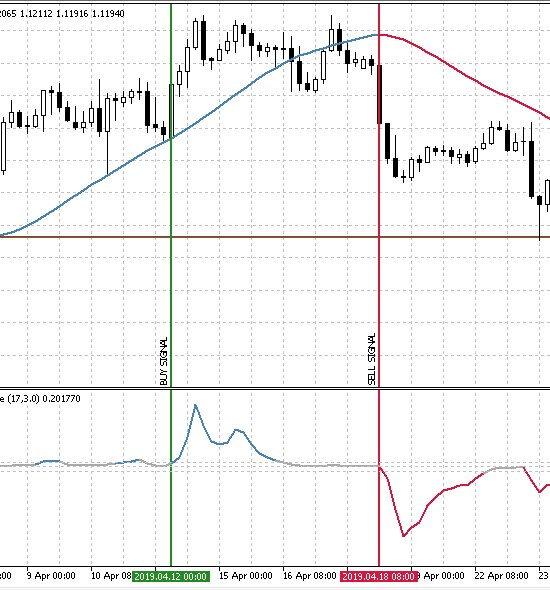### 测试价格速度测量方法

• 时间段: 近一年. (在写这篇文章时, 这是 01.01.2019 — 26.05.2019)
• 交易品种: EURUSD.
• 交易模式: 没有延迟 (这些不是高频交易策略， 所以延迟的影响将非常小).
• 测试: М1 OHLC (在真实分时上的预先测试显示了近似相同的结果).
• 初始存款: 1000 USD.
• 杠杆: 1:500.
• 服务器: MetaQuotes-Demo.
• 报价: 5-digit.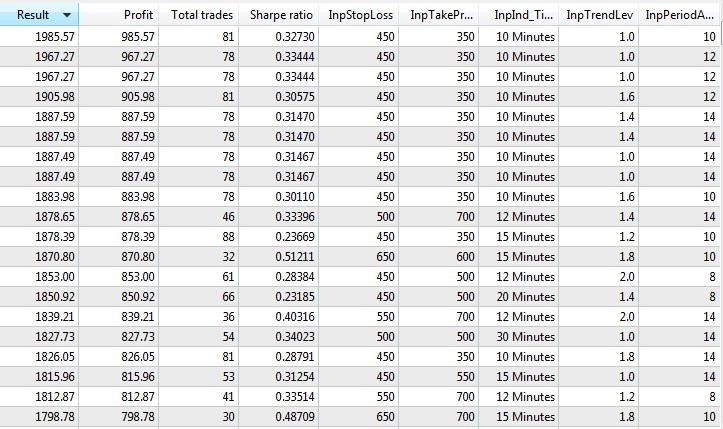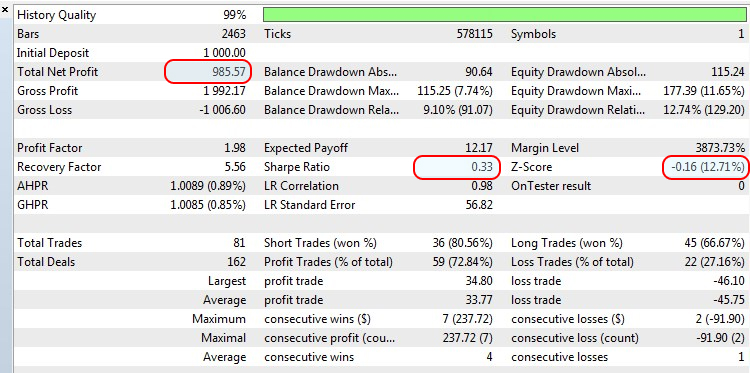• 测试: 把 M1 OHLC (在真实分时中的预先测试显示几乎相同的结果) 替换为 "基于真实分时的每一分时"。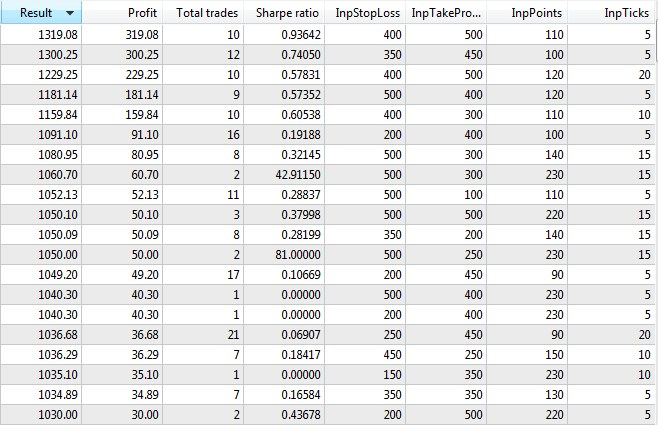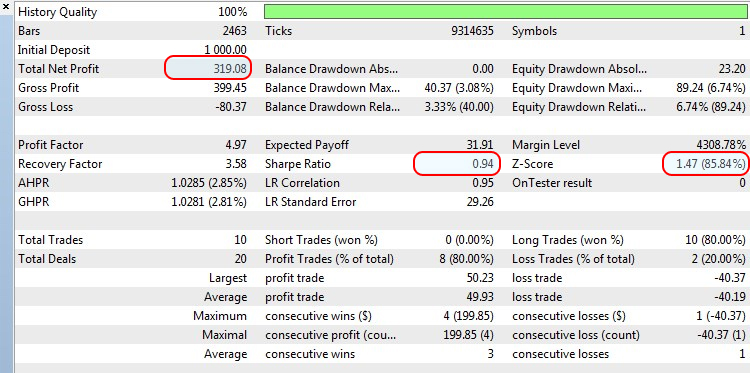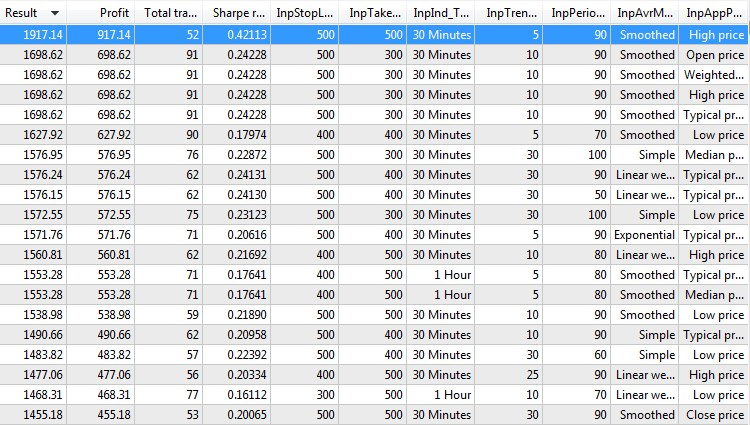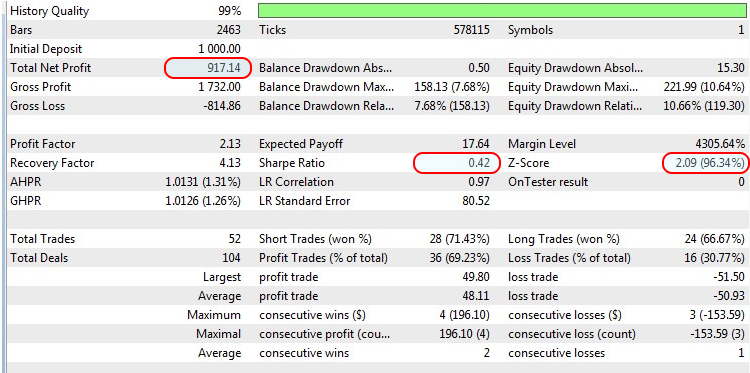### 结论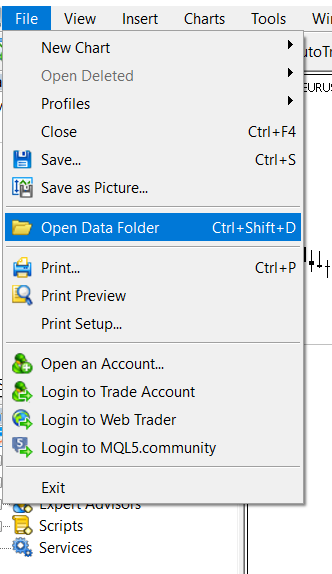#
名称

1
Strategy_1.mq5 EA  基于交易策略1的EA交易
2 Strategy_2.mq5 EA  基于交易策略2的EA交易
3 Strategy_3.mq5 EA   基于交易策略3的EA交易
4 Engine.mqh  交易函数库
5 平均速度  指标   在交易策略1中使用
6 CAM  指标    在交易策略1中使用
7 趋势方向和力度  指标    在交易策略3中使用
8 HMA_color  指标   在交易策略3中使用

MQL5.zip (734.69 KB)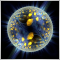评估分形指数和Hurst指数预测金融时间序列的能力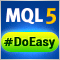轻松快捷开发 MetaTrader 程序的函数库(第七部分): StopLimit 订单激活事件，为修改订单和持仓事件准备功能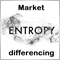通过差异化和熵值分析来探索市场"记忆"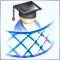开发跨平台网格EA(第二部分):在趋势方向上的基于范围的网格